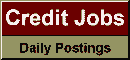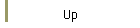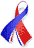DefaultRisk.com the web's biggest credit risk modeling resource.doi> search: A or B Export citation to:- HTML- Text (plain)- BibTeX- RIS- ReDIF

Linear Correlation Estimation

by Filip Lindskog of ETH-Zentrum

December 11, 2000

Abstract: Most financial models for modelling dependent risks are based on the assumption of multivariate normality and linear correlation is used a a measure of dependence. However, observed financial data are rarely normally distributed and tend to have marginal distributions with heavier tails. Furthermore, the observed synchronized extreme falls in financial markets can not be modelled by multivariate normal distributions. However, there are other elliptical distributions with these properties and the assumption of multivariate normality can often be replaced by the assumption of ellipticality. A useful property of elliptical distributions is that these distributions support the standard approaches of risk management. Value-at-Risk fulfills the desired properties of a risk measure and the mean-variance (Markowitz) approach can be u ed for portfolio optimization.

For elliptical distributions linear correlation is still a natural measure of dependence. However, a linear correlation estimator such a the Pearson product-moment correlation estimator (the standard estimator) being suitable for data from uncontaminated multivariate normal distributions ha a very bad performance for heavier tailed or contaminated data. Therefore robust estimator are needed, robust in the sense of being insensitive to contamination and yet maintaining a high efficiency for heavier tailed elliptical distributions a well a for multivariate normal distributions.

In this paper an overview of techniques for robust linear correlation estimation is given and the various techniques are compared for contaminated and uncontaminated elliptical distributions. In particular the relation

t = (2 / p) arcsin r

between Kendall's tau and the linear correlation coefficient r is shown to hold for (essentially) all elliptical distributions and the estimator of linear correlation provided by this relation is studied. This non-parametric estimator inherit the robustness properties of the Kendall's tau estimator and is an efficient (low variance) estimator for all elliptical distributions.

Books Referenced in this paper:  (what is this?)